# LeNet, 1998Fig. Structure of LeNet-5Fig. Another structure of LeNet-5
`````` {
"input"    : "a 32x32 picture",
"output"   : "10 classes [0~9]",
"database" : {
"name"    :"MNIST",
"img_size":"28x28",
},
"training" : {
"weighting" : ["kernel","bias"],
"method"    : "backpropagation"
},
"structure":{
"conv-layer"      : 3,
"pooling-layer"   : 2,
"full-connected"  : 1
}
}
``````

## Basic method

### A Convolution Layer

``````"kernel" : {
"kernel_size"       : "5x5",
"activated_method"  : "Sigmoid"
},
"param" : {
"kernel" : 25,
"bias"   : 1,
"total"  : 26
}
``````Fig. Activated Method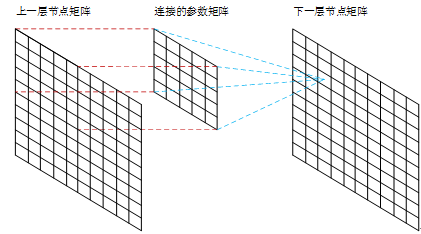Fig. Mapping Method

This means a feature map shares same weighting cuz they're using same kernel.

### A Pooling Layer (Sampling)

``````pooling: {
"method": "Mean-Pooling"
}
``````Fig. Method of Pooling

### Data Normalization

``````{
"white": -0.1,
"black": 1.175,
"reason" : "This makes the mean input roughly 0, and the variance roughly 1 which accelerates learning"
}
``````

## Analysis of each layer

### Conv1Fig. Structure of C1

``````"basic"{
"input"  : { "size":"32x32", "type": "img", "number": 1 },
"output" : { "size":"28x28", "type": "img", "numbers": 6 }
},
"feature_map" : {
"kernel_size"       : "5x5",
"activated_method"  : "Sigmoid",
"quantity"          : 6,
"param"             : "(5x5+1)x6 = 156"
},
"layer"{
"connected": "(5x5+1)x(28x28)x6 = 122304"
}
``````

### Pooling2Fig. Structure of S2

``````"basic"{
"input"  : { "size":"28x28", "type": "img", "numbers": 6 },
"output" : { "size":"14x14", "type": "img", "numbers": 6 }
},
"kernel_size"       : "2x2",
"activated_method"  : "Unknown",
"quantity"          : 1,
"param"             : "(weighting,bias)x6 = 12"
},
"layer"{
"connected": "5x14x14x6=5880"
}
``````

### Conv3

Convolution method is same as C1. The difference is a node in C3 connects with numbers of maps with S2 as shown below. This asymmetric linking is good to extract more combination of features.Fig.Structure of C3

Table. Connecting relation between S2 and C3 with the rule divided by red bounding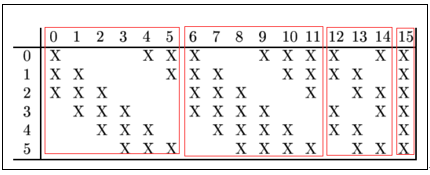A non-complete connection scheme keeps the number of connections within reasonable bounds.
More important, it forces a break of symmetry in the network.
Different feature amps are forced to extract different (hopefully complementary) features beacuse they get different sets of input.

``````"basic"{
"input"  : { "size":"14x14", "type": "img", "numbers": 6 },
"output" : { "size":"10x10", "type": "img", "numbers": 16 }
},
"feature_map" : {
"kernel_size"       : "5x5",
"activated_method"  : "Sigmoid",
"quantity"          : 16,
"param"             : "(5x5x3+1)x6 + (5x5x4 + 1) x 3 + (5x5x4 +1)x6 + (5x5x6+1)x1 = 1516"
},
"layer"{
"connected": "1516x10x10 = 151600"
}
``````

### Pooling4Fig.Structure of S4 (same method as S2)

``````"basic"{
"input"  : { "size":"10x10", "type": "img", "numbers": 16 },
"output" : { "size":"5x5", "type": "img", "numbers": 16 }
},
"kernel_size"       : "2x2",
"activated_method"  : "Unknown",
"quantity"          : 1,
"param"             : "(weighting,bias)x16 = 32"
},
"layer"{
"connected": "5x5x5x16=2000"
}
``````

### Conv5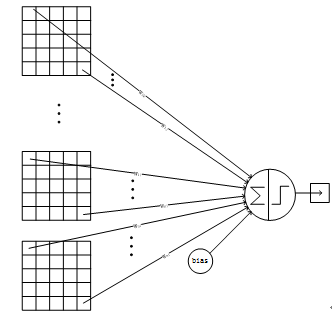Fig.Structure of C5

``````"basic"{
"input"  : { "size":"5x5", "type": "img", "number": 16 },
"output" : { "size":"1x1", "type": "img", "numbers": 120 }
},
"feature_map" : {
"kernel_size"       : "5x5",
"activated_method"  : "Sigmoid",
"quantity"          : 120,
"param"             : "(5x5x16+1)x120 = 48120"
},
"layer"{
"connected": "(5x5x16+1)x120 = 48120"
}
``````

### F6 (Full-connected)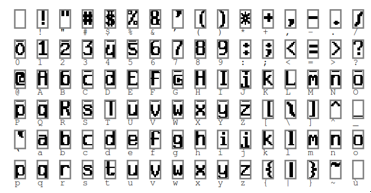Fig. Bits map of codes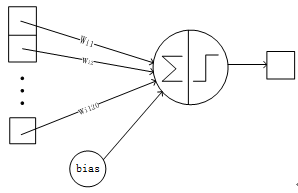Fig. Structure of Full-connected layer f6

Full-connected Layer (f6) has 84 nodes, mapping to the bits map of codes (7x12).
This means a 1x1 on bits map is a output of the F6, and 84 ouput form the whole bits map.

``````"basic"{
"input"  : { "size":"1x1", "type": "img", "number": 120 },
"output" : { "size":"1x1", "type": "number", "data":{"white":-1,"black":1}, "numbers": 84 }
},
"activation" : {
"activated_method"  : "Sigmoid",
"param"             : "(120+1)x84 = 10164"
},
"layer"{
"connected": "(120+1)x84 = 10164"
}
``````

### Output Layer

Output Layer is a full-connected layer with 10 nodes (0~9)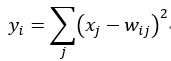Fig. RBF output function

Where y is output of RBF, x is input from previos layer.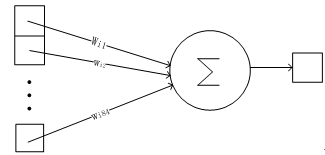Fig. Structure of Output layer
Full-connected Layer (f6) has 84 nodes, mapping to the bits map of codes (7x12). This means a 1x1 on bits map is a output of the F6, and 84 ouput form the whole bits map.

``````"basic"{
"input"  : { "size":"1x1", "type": "number", "data":{"white":-1,"black":1}, "number": 84 },
"output" : { "size":"1x1", "type": "number","data":{"number":[0~9]}, "numbers": 10 }
},
"activation" : {
"activated_method"  : "Sigmoid",
"param"             : "84x10 = 840"
},
"layer"{
"connected": "84x10 = 840"
}
``````

### Result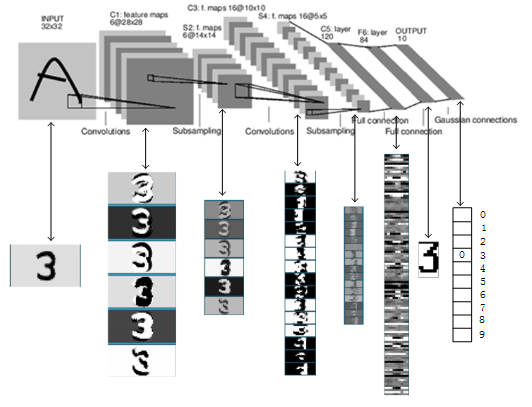Fig. Process of LeNet-5 recognizing "3"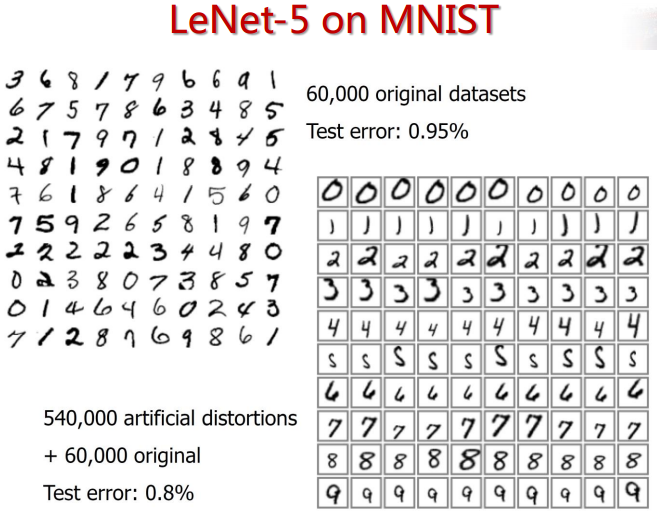Fig. Experimental Result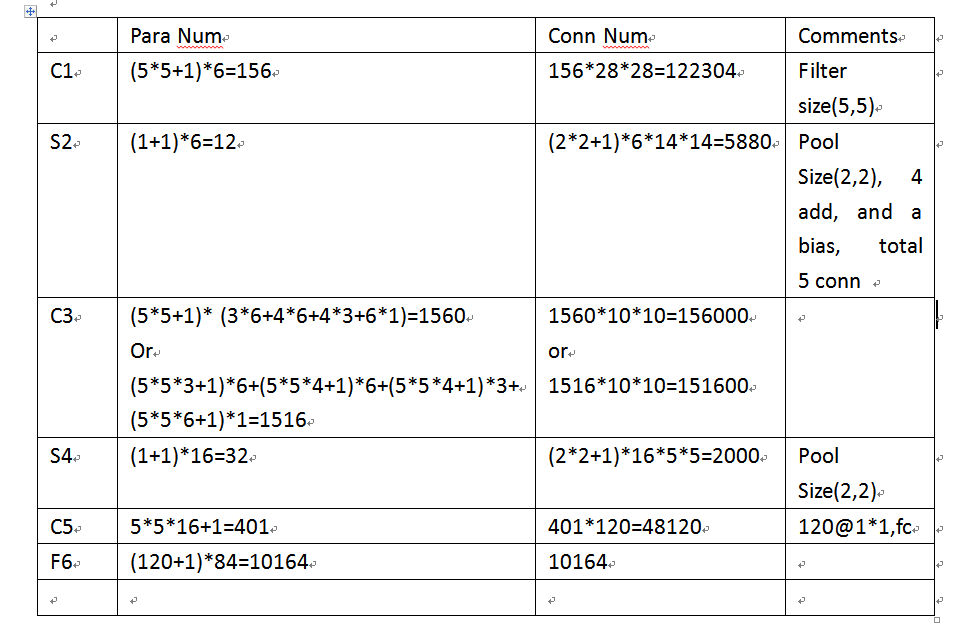Fig. Total Parameter Table

``````"basic"{
"input"  : { "size":"32x32", "type": "img", "number": 1 },
"output" : { "size":"1x1", "type": "class","data":{"number":[0~9]}, "numbers": 10 }
},
"training" : {
"param" : "60,840"
},
"layer"{
"connected": "340,908"
}
``````

[ REFERENCE ]

 Y. LeCun, L. Bottou, Y. Bengio, and P. Haffner. Gradient-based learning applied to document recognition Proceedings of the IEEE, Nov. 1998.